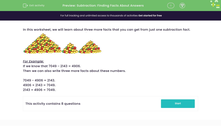# Use a Subtraction Fact to Find Other Number Facts

In this worksheet, students will learn that addition and subtraction are inverses and that you can find other facts from just one subtraction fact.Key stage:  KS 2

Curriculum topic:   Number: Addition and Subtraction

Curriculum subtopic:   Add/Subtract up to Four-Digit Numbers

Difficulty level:#### Worksheet Overview

In this activity, we will be learning about three more facts that you can get from just one subtraction fact.Example:

We know that 7,049 - 2,143 = 4,906

So, we can write three more facts about these numbers.

7,049 - 4,906 = 2,143
4,906 + 2,143 = 7,049
2,143 + 4,906 = 7,049

Here is an easier example:

If we know that 100 - 30 = 70, then we can also work out the following:

100 - 70 = 30

70 + 30 = 100

30 + 70 = 100

Let's practise spotting these addition and subtraction facts in the questions that follow!### What is EdPlace?

We're your National Curriculum aligned online education content provider helping each child succeed in English, maths and science from year 1 to GCSE. With an EdPlace account you’ll be able to track and measure progress, helping each child achieve their best. We build confidence and attainment by personalising each child’s learning at a level that suits them.

Get started